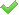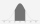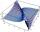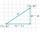Hyperbola

Find the equation of hyperbola that passes through the point M [30; 24] and has focal points at F1 [0; 4 sqrt 6], F2 [0; -4 sqrt 6].

Result

h = (Correct answer is: )Solution:Leave us a comment of this math problem and its solution (i.e. if it is still somewhat unclear...):Be the first to comment!To solve this verbal math problem are needed these knowledge from mathematics:

Looking for help with calculating arithmetic mean? For Basic calculations in analytic geometry is helpful line slope calculator. From coordinates of two points in the plane it calculate slope, normal and parametric line equation(s), slope, directional angle, direction vector, the length of segment, intersections the coordinate axes etc. Looking for a statistical calculator? Pythagorean theorem is the base for the right triangle calculator.

Next similar math problems:Given that P = (5, 8) and Q = (6, 9), find the component form and magnitude of vector PQ.
2. Find the 3Find the distance and mid-point between A(1,2) and B(5,5).
3. 75th percentile (quartille Q3)Find 75th percentile for 30,42,42,46,46,46,50,50,54
4. Vector 7Given vector OA(12,16) and vector OB(4,1). Find vector AB and vector |A|.
5. Median and modusRadka made 50 throws with a dice. The table saw fit individual dice's wall frequency: Wall Number: 1 2 3 4 5 6 frequency: 8 7 5 11 6 13 Calculate the modus and median of the wall numbers that Radka fell.
6. Center of line segmentCalculate the distance of the point X [1,3] from the center of the line segment x = 2-6t, y = 1-4t ; t is .
7. DistanceWha is the distance between the origin and the point (18; 22)?
8. Theorem proveWe want to prove the sentence: If the natural number n is divisible by six, then n is divisible by three. From what assumption we started?
9. ABS CNCalculate the absolute value of complex number -15-29i.
10. Euclid2In right triangle ABC with right angle at C is given side a=27 and height v=12. Calculate the perimeter of the triangle.
11. Unit vector 2DDetermine coordinates of unit vector to vector AB if A[-6; 8], B[-18; 10].
12. The ditchDitch with cross section of an isosceles trapezoid with bases 2m 6m are deep 1.5m. How long is the slope of the ditch?
13. Triangle IRTIn isosceles right triangle ABC with right angle at vertex C is coordinates: A (-1, 2); C (-5, -2) Calculate the length of segment AB.
14. AverageIf the average(arithmetic mean) of three numbers x,y,z is 50. What is the average of there numbers (3x +10), (3y +10), (3z+10) ?
15. Life expectancyThe life expectancy of batteries has a normal distribution with a mean of 350 minutes and standard deviation of 10 minutes. What the range in minutes 68% of the batteries will last? What is the range in minutes approximately 99.7% of batteries will last?
16. Harmonic and arithmetic meansThe local Utah Department of Child Service office wants to project staffing needs based on current social worker assignments. They have the number of cases per social worker for the following staff: Mary: 25 John: 35 Ted: 15 Lisa: 45 Anna: 20 Calculat
17. CenterCalculate the coordinates of the center of gravity T [x, y] of triangle ABC; A[11,4] B[13,-7] C[-17,-18].